# Balian-Low theorem

A theorem dealing with the representation of arbitrary functions in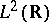as a sum of time-frequency atoms, or Gabor functions (cf. also Gabor transform), of the form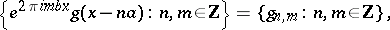where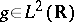is a fixed window function and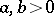are fixed lattice parameters. The goal is to write an arbitrary function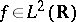in a series of the form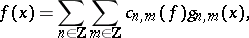(a1)

where the coefficients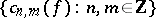depend linearly on. One requires that the collection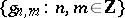forms a frame for, that is, that there exist constants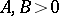such that for any,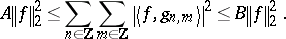(a2)

Inequality (a2) implies the existence of coefficientssatisfying (a1) and the inequality. This inequality can be interpreted as expressing the continuous dependence ofon the coefficientsand the continuous dependence of these coefficients on. Whether or not an arbitrary collection of Gabor functionsforms a frame fordepends on the window function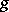and on the lattice density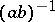. The lattice density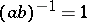is referred to as the critical density, for the following reason. Ifandforms a frame, then that frame is non-redundant, i.e., it is a Riesz basis. If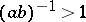andforms a frame, then that frame is redundant, i.e., the representation (a1) is not unique. If, then for any, the collectionis incomplete. See [a9], [a10].

The time-frequency atom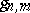is said to be localized at time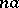and frequency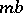since the Fourier transform ofis given by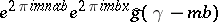. A window functionis said to have "good localization" in time and frequency if bothand its Fourier transform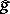decay rapidly at infinity. Good localization can be measured in various ways. One way is to require that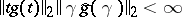. This is related to the classical uncertainty principle inequality, which asserts that any functionsatisfies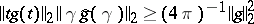.

The Balian–Low theorem asserts that ifand ifforms a frame for, thencannot have good localization. Specifically: Ifand ifforms a frame for, then, i.e.,maximizes the uncertainty principle inequality.

More generally, the term "Balian–Low theorem" or "Balian–Low-type theorem" can refer to any theorem which asserts time and frequency localization restrictions on the elements of a Riesz basis. Such theorems include, for example, [a4], Thm. 3.2, in which a different criterion for "good localization" for the elements of a Gabor system is used, [a7], Thm. 4.4, in which more general time-frequency lattices for Gabor systems are considered, and [a3], which asserts a time-frequency restriction on bases of wavelets (cf. also Wavelet analysis).

The Balian–Low theorem was originally stated and proved by R. Balian [a1] and independently by F. Low [a8] under the stronger assumption thatforms an orthonormal basis (cf. also Orthonormal system) for, and an extension of their argument to frames was given by I. Daubechies, R.R. Coifmann and S. Semmes [a5]. An elegant and entirely new proof of the theorem for orthonormal bases using the classical uncertainty principle inequality was given by G. Battle [a2], and an extension of this argument to frames was given by Daubechies and A.J.E.M. Janssen [a6]. Proofs of the Balian–Low theorem for frames use the differentiability properties of the Zak transform in an essential way.